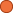## ← Blueberries for friends

• 2 Followers
• 104 Lines

### Get Embed Code x Embed video Use the following code to embed this video. See our usage guide for more details on embedding. Paste this in your document somewhere (closest to the closing body tag is preferable): ```<script type="text/javascript" src='https://amara.org/embedder-iframe'></script> ``` Paste this inside your HTML body, where you want to include the widget: ```<div class="amara-embed" data-url="http://www.youtube.com/watch?v=QXNg_u5Tv8Q" data-team="null"></div> ``` 10 Languages

•английски език [en] оригинал
•чешки език [cs]
•индонезийски език [id]
•италиански език [it]
•грузински език [ka]
•Корейски [ko]
•холандски [nl] незавършен
•тамил [ta]
•украински език [uk]
•Chinese, Simplified [zh-cn]

Showing Revision 3 created 08/25/2015 by Chockkalingam Karuppaiah.

1. Kali is having a picnic for her six friends.
2. The oldest friend, Vikram, is 10 years old.
3. The youngest, Diya, is six years old.
4. She has a total of 48 blueberries
5. and wants to split them evenly
6. between her friends.
7. How many blueberries does each friend get?
8. And I encourage you to now,
9. pause this video
10. and try to figure it out on your own.
11. How many blueberries does each friend get?
13. So she has six friends.
14. She has six friends
15. and she wants them all
16. to be able to get the same amount.
17. So, she wants to split the 48 blueberries evenly
18. amongst her six friends.
19. The ages of her friends don't matter.
20. So, she's going to take the 48 blueberries.
21. She's going to take the 48 blueberries
22. and divide it,
23. and divide it
24. by six.
25. She wants to divide it into six groups.
26. So she wants to divide it into six groups.
27. 48 divided by 6.
28. And so this,
29. so question mark,
30. is going to be equal to the number of blueberries
31. that each friend gets.
32. So 48 divided by six is equal to question mark,
33. is the same thing as saying
34. that 48,
35. 48 is equal to,
36. is equal to question mark times six,
37. times six.
38. So if we could figure out
39. what number we can multiply by six
40. to get 48,
41. then we know what 48 divided by six
42. actually is.
43. For example, this question mark,
44. this is the number of blueberries per friend.
45. The number of blueberries per friend times six friends,
46. well that should tell us the total number of blueberries,
47. which is 48.
48. So what is this number?
51. all of the multiples of six.
52. So, six times one is equal to six.
53. Six times two is equal to 12.
54. And really we're just increasing by six each time.
55. We're just increasing by six.
56. Six times three is equal to 18.
57. Six times four is equal to 24.
58. Six times five is equal to 30.
59. Six times six is equal to 36.
60. Six times seven is equal to 42.
61. Notice we're just adding six every time.
62. Six times eight is equal to 48.
63. Is equal to 48.
64. So we now know that question mark,
65. we now know that the question mark must be eight.
66. Six times eight
67. and eight times six is the same thing.
68. So this is going to be equal
69. to six times question mark.
70. Six times question mark
71. and now we learned
72. that question mark is equal to 48.
73. Sorry, question mark is equal to eight.
74. So, each of her friends
75. are going to get eight blueberries.
76. So this is, right over here,
77. 48 divided by six is equal to eight blueberries.
78. Now, let's verify that.
79. We have 48 blueberries right over here.
80. Lets divide them into six groups
81. where each group is going to have eight blueberries.
82. So let me do my best.
83. So let's see, this is one, two,
84. three, four, five, six, seven, eight.
85. So, that's a group of eight blueberries.
86. See, here's another group of eight blueberries,
87. right over here.
88. And there's another group, right over here,
89. of eight blueberries.
90. And then, here's our fourth group
91. of eight blueberries.
92. And now, let's see,
93. we can make a fifth group
94. of eight blueberries.
95. Fifth group of eight blueberries.
96. And then, finally,
97. here is our sixth group.
98. Here is our sixth group
99. of eight blueberries.
100. So notice,
101. we have six groups of eight blueberries.
102. Each of her six friends
103. could take one of these groups
104. of eight blueberries.